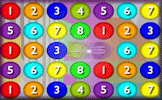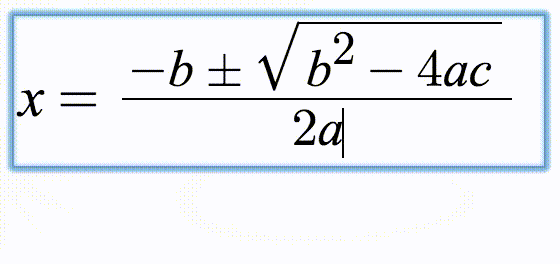# Fractionagons

## Calculate the missing fractions in these partly completed arithmagon puzzles.

##### MenuArithmagonsLevel 1Level 2Level 3Level 4Level 5HelpMore Fractions

Complete the fractionagons so that the fractions in the rectangles are the sums of the fractions at the vertices of the triangles. Use the forward slash / to type a fraction. Give answers in their lowest terms.

 $$\frac{1}{8}$$ $$\frac{3}{8}$$ $$\frac{1}{4}$$+ $$\frac{3}{10}$$ $$\frac{1}{2}$$ $$\frac{1}{10}$$+ $$\frac{3}{7}$$$$\frac{5}{14}$$ $$\frac{4}{7}$$+ $$\frac{1}{3}$$ $$\frac{4}{9}$$$$\frac{2}{3}$$+ $$\frac{1}{4}$$$$\frac{3}{4}$$$$\frac{3}{8}$$ +$$\frac{5}{12}$$ $$\frac{1}{2}$$ $$\frac{7}{12}$$+
Check

This is Fractionagons level 1. You can also try:
Level 2 Level 3 Level 4 Level 5

## Instructions

Try your best to answer the questions above. Type your answers into the boxes provided leaving no spaces. As you work through the exercise regularly click the "check" button. If you have any wrong answers, do your best to do corrections but if there is anything you don't understand, please ask your teacher for help.

When you have got all of the questions correct you may want to print out this page and paste it into your exercise book. If you keep your work in an ePortfolio you could take a screen shot of your answers and paste that into your Maths file.

## Transum.org

This web site contains over a thousand free mathematical activities for teachers and pupils. Click here to go to the main page which links to all of the resources available.## More Activities:

Mathematicians are not the people who find Maths easy; they are the people who enjoy how mystifying, puzzling and hard it is. Are you a mathematician?

Comment recorded on the 21 October 'Starter of the Day' page by Mr Trainor And His P7 Class(All Girls), Mercy Primary School, Belfast:

"My Primary 7 class in Mercy Primary school, Belfast, look forward to your mental maths starters every morning. The variety of material is interesting and exciting and always engages the teacher and pupils. Keep them coming please."

Comment recorded on the 5 April 'Starter of the Day' page by Mr Stoner, St George's College of Technology:

"This resource has made a great deal of difference to the standard of starters for all of our lessons. Thank you for being so creative and imaginative."

Each month a newsletter is published containing details of the new additions to the Transum website and a new puzzle of the month.

The newsletter is then duplicated as a podcast which is available on the major delivery networks. You can listen to the podcast while you are commuting, exercising or relaxing.

Transum breaking news is available on Twitter @Transum and if that's not enough there is also a Transum Facebook page.

#### Number Crunch SagaA lively numeracy game requiring you to align three numbers to create the given target sum or product. There are five levels to this online game and a virtual Transum Trophy available for each level.

There are answers to this exercise but they are available in this space to teachers, tutors and parents who have logged in to their Transum subscription on this computer.

A Transum subscription unlocks the answers to the online exercises, quizzes and puzzles. It also provides the teacher with access to quality external links on each of the Transum Topic pages and the facility to add to the collection themselves.

Subscribers can manage class lists, lesson plans and assessment data in the Class Admin application and have access to reports of the Transum Trophies earned by class members.

Subscribe

## Go Maths

Learning and understanding Mathematics, at every level, requires learner engagement. Mathematics is not a spectator sport. Sometimes traditional teaching fails to actively involve students. One way to address the problem is through the use of interactive activities and this web site provides many of those. The Go Maths page is an alphabetical list of free activities designed for students in Secondary/High school.

## Maths Map

Are you looking for something specific? An exercise to supplement the topic you are studying at school at the moment perhaps. Navigate using our Maths Map to find exercises, puzzles and Maths lesson starters grouped by topic.

## Teachers

If you found this activity useful don't forget to record it in your scheme of work or learning management system. The short URL, ready to be copied and pasted, is as follows:

Alternatively, if you use Google Classroom, all you have to do is click on the green icon below in order to add this activity to one of your classes.

It may be worth remembering that if Transum.org should go offline for whatever reason, there is a mirror site at Transum.info that contains most of the resources that are available here on Transum.org.

When planning to use technology in your lesson always have a plan B!

Saturday, June 18, 2022

Do you have any comments? It is always useful to receive feedback and helps make this free resource even more useful for those learning Mathematics anywhere in the world. Click here to enter your comments.For All:

© Transum Mathematics :: This activity can be found online at:
www.Transum.org/go/?Num=890

## Description of Levels

CloseArithmagons - The same sort of puzzle but with numbers not algebra.

Level 1 - Sums of simple fractions

Level 2 - Sums of fractions where one denominator is not a multiple of the other.

Level 3 - Sums of mixed numbers.

Level 4 - Multiplication of fractions.

Level 5 - Multiplication of mixed numbers.

Algebragons - Different topic, same structure.

More Fractions including lesson Starters, visual aids, investigations and self-marking exercises.

Answers to this exercise are available lower down this page when you are logged in to your Transum account. If you don’t yet have a Transum subscription one can be very quickly set up if you are a teacher, tutor or parent.

## Mathematical Notation

Use the forward slash / to type a fraction then the right arrow or tab key to end the fraction.

For example: Type 1/2 to get ½.

Fractions should be given in their lowest terms.

Don't wait until you have finished the exercise before you click on the 'Check' button. Click it often as you work through the questions to see if you are answering them correctly. You can double-click the 'Check' button to make it float at the bottom of your screen.

Answers to this exercise are available lower down this page when you are logged in to your Transum account. If you don’t yet have a Transum subscription one can be very quickly set up if you are a teacher, tutor or parent.

Here are some suggestions for how you may solve the puzzles in which only the three values in the rectangles are given (the last question in levels three and above):

### For novice learners

1. Guess a value for the top circle
2. From that calculate the values that would be in the bottom two circles
3. Do these two values sum to the bottom rectangle? If so finish.
4. If not is their sum too high or too low? Adjust the top circle value accordingly and go back to (2)

Replace 'sum' with 'product' if the operation is multiplication.

1. Let x stand for the top circle value
2. From that find expressions for the bottom two circles
3. Make an equation from the bottom two circles and the bottom rectangle
4. Solve that equation.

### A great 'trick'

This was found by Ann Roberts, a Transum subscriber, who wrote with this method of solving an arithmagon with 18, 15 and 11 in the rectangles. It also works for fractions.

1. Add the numbers in the rectangles: 18 + 15 + 11 = 44. This is the same as adding the numbers in the blank circles twice.
2. Divide this sum by two: 44 ÷ 2 = 22. This is now the same as adding the numbers in the blank circles once.
3. Finally: 15 is the sum of the bottom white circles so 15 + top white circle = 22

### Typing Mathematical Notation

These exercises use MathQuill, a web formula editor designed to make typing Maths easy and beautiful. Watch the animation below to see how common mathematical notation can be created using your keyboard.Close